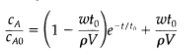### Create an Account

Home / Questions / Irreversible first order reaction in a continuous reactor a well stirred reactor of volume...

# Irreversible first order reaction in a continuous reactor a well stirred reactor of volume V is initially completely filled with a solution of solute A in a solvent S at concentration CAO At time

Irreversible first-order reaction in a continuous reactor, a well-stirred reactor of volume V is initially completely filled with a solution of solute A in a solvent S at concentration CAO. At time t = 0, an identical solution of a in S is introduced at a constant mass flow rate w. A small constant stream of dissolved catalyst is introduced at the same time, causing A to disappear according to an irreversible first-order reaction with rate constant k'''1 sec the rate constant may be assumed independent of composition and time. Show that the concentration of A in the reactor (assumed isothermal) at any time is in which t0 = [(w/pV) +k'''1].View less »

Jul 25 2020 View more View LessSubscribe To Get Solution